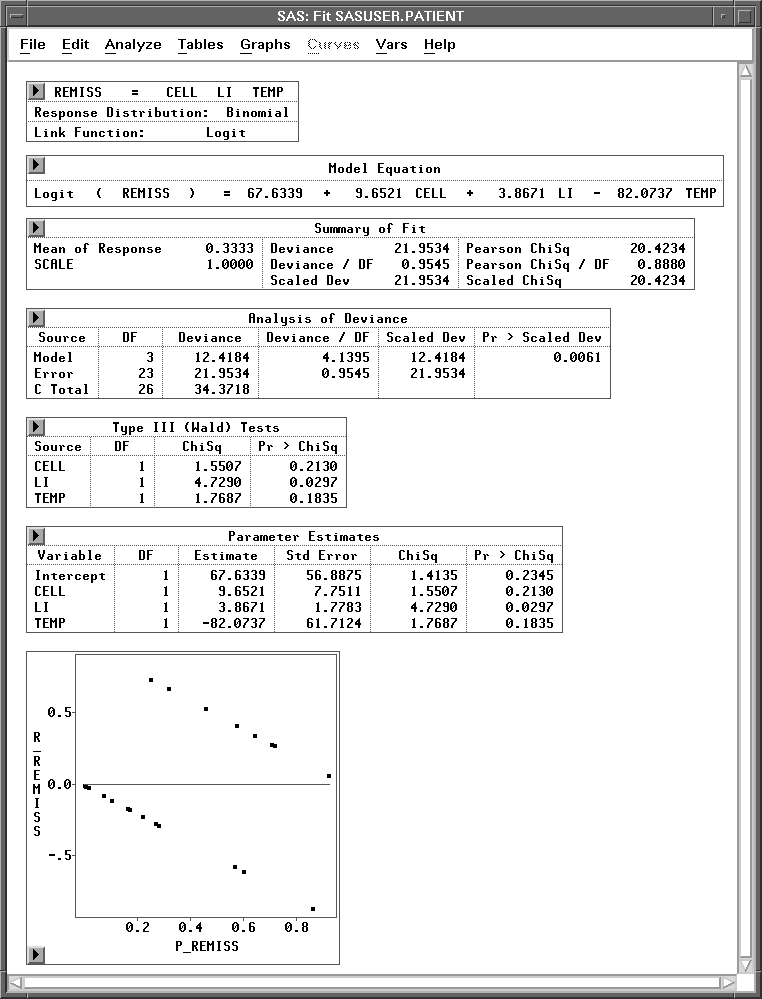Previous Page Next Page
 Logistic Regression

# Logistic Regression

In the last two chapters, you used least-squares methods to fit linear models. In this chapter, you use maximum-likelihood methods to fit generalized linear models. You can choose Analyze:Fit ( Y X ) to carry out a logistic regression analysis. You can use the fit variables and method dialogs to specify generalized linear models and to add and delete variables from the model.Figure 16.1: Logistic Regression Analysis

#### References

 Previous Page Next Page Top of Page